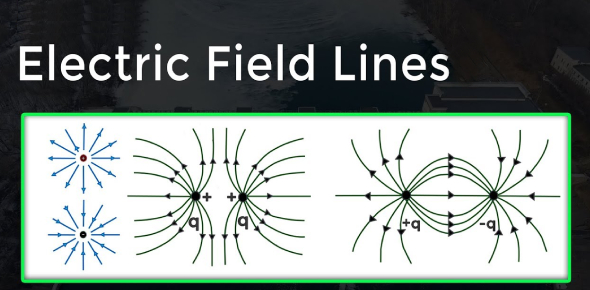# Electric Field Lines Quiz

13 Questions | Total Attempts: 3026Settings.

• 1.
Field lines start on positive charges and end on negative charges.
• A.

True

• B.

False

• 2.
When drawing field lines, the lines should always cross.
• A.

True

• B.

False

• 3.
Electric field lines are used to describe the electric field around a charged object.
• A.

True

• B.

False

• 4.
When two equally charged plates are placed over one another the field lines between them are completely straight and not evenly spaced.
• A.

True

• B.

False

• 5.
Some organisms produce electric fields.
• A.

True

• B.

False

• 6.
In electrostatic representations electric field lines start on ________ charges and end on _______ charges.
• 7.
The electric field is _____ at the midpoint of two equally charged particles.
• 8.
The magnitude of the electric field between two equally charged plates is directly ___________ to the charge per unit.
• 9.
When a test charge is placed between two equally charged plates the charge is _______ by the upper plate and _________ to the lower plate.
• 10.
The number of lines leaving a positive charge or approaching a negative charge is proportional to the _________ of the charge.
• 11.
Name an animal in nature who would use electric fields to survive in nature. How would they use this field?
• 12.
In the diagram below which particle has a negative charge and which one has a positive charge? Why?
• 13.
Draw the field lines between two equally charged plates.
Related TopicsBack to top